# Line

The Line command draws one line segment.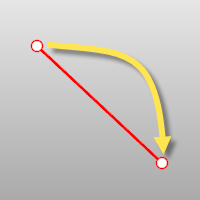Steps

 1 Pick the start of the line.
 2 Pick the end of the line. Use object snaps to reference existing geometry.

Command-line options

BothSides

The BothSides option draws the object on both sides of the start point, creating the object twice as long as you indicate.The BothSides option demonstrated with the Line command.

Normal

The Normal option draws the line normal to a location on a surface.Normal steps

 1 Select a surface.
 2 Pick the start of the line on the surface.
 3 Pick the end of the line or type a length, and press Enter.

Normal option

IgnoreTrims=Yes/No

If Yes, surface trims are ignored. When the marker misses the untrimmed surface, the no-access cursor is shown.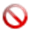No access cursor.

Angled

The Angled option draws the line at a specified angle from a reference line.Angled steps

 1 Pick the start of a base (reference) line.
 2 Pick the end of a base (reference) line.
 3 Type the pivot angle, and press Enter.
 4 Pick the end of the line.

Vertical

The Vertical option draws the line vertical to the construction plane.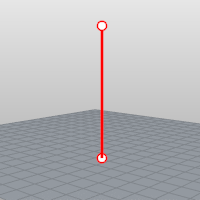Vertical steps

 1 Pick the start of the line.
 2 Pick the end of the line or type a length and press Enter.

FourPoint

The FourPoint option draws the line using two points to establish direction and two points to establish length.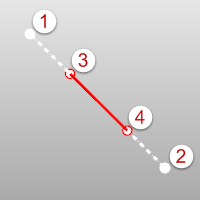FourPoint steps

 1 Pick the start of the base line (reference location).
 2 Pick the end of the base line (second reference location).
 3 Pick the start of the line.
 4 Pick the end of the line.

Bisector

The Bisector option draws the line that bisects a specified angle.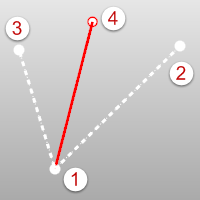Bisector steps

 1 Pick the start of the bisector line.
 2 Pick the start of the angle to bisect.
 3 Pick the end of the angle to bisect.
 4 Pick the end of the line or type a length, and press Enter.

Perpendicular

The Perpendicular option draws the line perpendicular to or from a curve.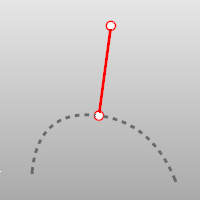Perpendicular steps

 1 Pick the start of the line on a curve.
 2 Pick the end of the line.

Perpendicular options

Point

The Point option allows you to pick a point that is near, but not on a curve, overriding the built-in object snap.FromFirstPoint

The FromFirstPoint option forces the line to go through the first picked point on the curve instead of allowing the point to slide along the curve.

2Curves

The 2Curves option restricts the line to be perpendicular to two curves.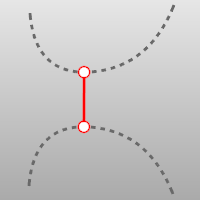Tangent

The Tangent option draws the line tangent from a curve.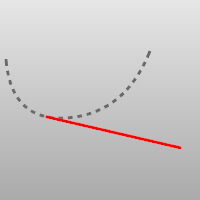Tangent steps

 1 Pick the start of the line on a curve.
 2 Pick the end of the line.

Tangent options

Point

The Point option allows you to pick a point that is near, but not on a curve, overriding the built-in object snap.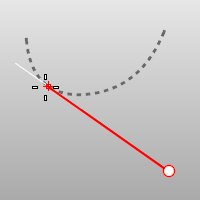FromFirstPoint

The FromFirstPoint option forces the line to go through the first picked point on the curve instead of allowing the point to slide along the curve.

2Curves

The 2Curves option restricts line to be tangent to two curves.Extension

Extends a curve with a line.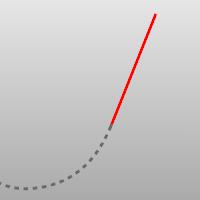Extension steps

 1 Select a curve (line) near the end you want to extend.
 2 Pick the end of the line or type a distance and press Enter.